# B.Tech. (SEM-II) THEORY EXAMINATION 2016-17 COMPUTER SYSTEM & PROGRAMMING IN C

Time: 3hrs
Total Marks: 100

Note:-

• There are three sections. Section A carries 20 marks, Section B carries 30 marks and Section C carries 50 marks.
• Attempt all questions. Marks are indicated against each question.
• Assume suitable data wherever necessary.

# Section – B

2. Attempt any five questions: (5*10 = 50)

a) i) What is the role of SWITCH statement in C programming language. Explain with example.
Switch case statements are a substitute for long if statements that compare a variable to several integral values

• The switch statement is a multiway branch statement. It provides an easy way to dispatch execution to different parts of code based on the value of the expression.
• Switch is a control statement that allows a value to change control of execution.

Syntax:

```switch (n)
{
case 1: // code to be executed if n = 1;
break;
case 2: // code to be executed if n = 2;
break;
default: // code to be executed if n doesn't match any cases
}```

Flowchart:Example:

 `// Following is a simple program to demonstrate ` `// syntax of switch. ` `#include ` `int` `main() ` `{ ` `    ``int` `x = 2; ` `    ``switch` `(x) { ` `    ``case` `1: ` `        ``printf``(``"Choice is 1"``); ` `        ``break``; ` `    ``case` `2: ` `        ``printf``(``"Choice is 2"``); ` `        ``break``; ` `    ``case` `3: ` `        ``printf``(``"Choice is 3"``); ` `        ``break``; ` `    ``default``: ` `        ``printf``(``"Choice other than 1, 2 and 3"``); ` `        ``break``; ` `    ``} ` `    ``return` `0; ` `} `

Output:

```Choice is 2
```

a) ii) What is recursion? Write a program in C to generate the Fibonacci series.
The process in which a function calls itself directly or indirectly is called recursion and the corresponding function is called as recursive function. Using recursive algorithm, certain problems can be solved quite easily. Examples of such problems are Towers of Hanoi (TOH), Inorder/Preorder/Postorder Tree Traversals, DFS of Graph, etc.

Base condition in recursion?
In recursive program, the solution to base case is provided and solution of bigger problem is expressed in terms of smaller problems.

```int fact(int n)
{
if (n < = 1) // base case
return 1;
else
return n*fact(n-1);
}
```

In the above example, base case for n < = 1 is defined and larger value of number can be solved by converting to smaller one till base case is reached.

Program in C to generate the Fibonacci series:

 `// Fibonacci Series using Recursion ` ` `  `#include ` ` `  `int` `fib(``int` `n) ` `{ ` `    ``if` `(n <= 1) ` `        ``return` `n; ` `    ``return` `fib(n - 1) + fib(n - 2); ` `} ` ` `  `int` `main() ` `{ ` `    ``int` `n = 9; ` `    ``printf``(``"%d"``, fib(n)); ` `    ``getchar``(); ` `    ``return` `0; ` `} `

Output:

```34
```

b) i) Differentiate between-

1. Actual and formal parameters:
• Formal Parameter : A variable and its type as they appear in the prototype of the function or method.
• Actual Parameter : The variable or expression corresponding to a formal parameter that appears in the function or method call in the calling environment.
2. global and extern variables
• As the name suggests, Global Variables can be accessed from any part of the program.
• They are available through out the life time of a program.
• They are declared at the top of the program outside all of the functions or blocks.
• Declaring global variables: Global variables are usually declared outside of all of the functions and blocks, at the top of the program. They can be accessed from any portion of the program.
• extern: Extern storage class simply tells us that the variable is defined elsewhere and not within the same block where it is used. Basically, the value is assigned to it in a different block and this can be overwritten/changed in a different block as well. So an extern variable is nothing but a global variable initialized with a legal value where it is declared in order to be used elsewhere. It can be accessed within any function/block. Also, a normal global variable can be made extern as well by placing the ‘extern’ keyword before its declaration/definition in any function/block. This basically signifies that we are not initializing a new variable but instead we are using/accessing the global variable only. The main purpose of using extern variables is that they can be accessed between two different files which are part of a large program. For more information on how extern variables work, have a look at this link.

b) ii) Describe about the basic components of a computer with a neat block diagram.
Digital Computer: A digital computer can be defined as a programmable machine which reads the binary data passed as instructions, processes this binary data, and displays a calculated digital output. Therefore, Digital computers are those that work on the digital data.

Details of Functional Components of a Digital Computer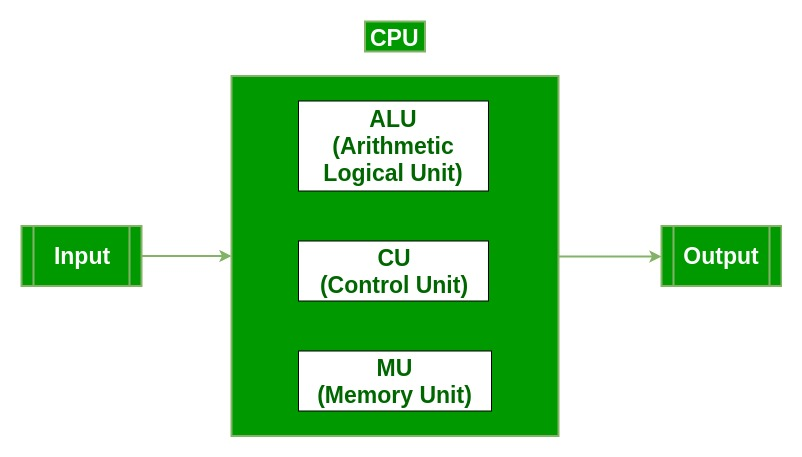• Input Unit :The input unit consists of input devices that are attached to the computer. These devices take input and convert it into binary language that the computer understands. Some of the common input devices are keyboard, mouse, joystick, scanner etc.
• Central Processing Unit (CPU0 : Once the information is entered into the computer by the input device, the processor processes it. The CPU is called the brain of the computer because it is the control center of the computer. It first fetches instructions from memory and then interprets them so as to know what is to be done. If required, data is fetched from memory or input device. Thereafter CPU executes or performs the required computation and then either stores the output or displays on the output device. The CPU has three main components which are responsible for different functions – Arithmetic Logic Unit (ALU), Control Unit (CU) and Memory registers
• Arithmetic and Logic Unit (ALU) : The ALU, as its name suggests performs mathematical calculations and takes logical decisions. Arithmetic calculations include addition, subtraction, multiplication and division. Logical decisions involve comparison of two data items to see which one is larger or smaller or equal.
• Control Unit : The Control unit coordinates and controls the data flow in and out of CPU and also controls all the operations of ALU, memory registers and also input/output units. It is also responsible for carrying out all the instructions stored in the program. It decodes the fetched instruction, interprets it and sends control signals to input/output devices until the required operation is done properly by ALU and memory.
• Memory Registers : A register is a temporary unit of memory in the CPU. These are used to store the data which is directly used by the processor. Registers can be of different sizes(16 bit, 32 bit, 64 bit and so on) and each register inside the CPU has a specific function like storing data, storing an instruction, storing address of a location in memory etc. The user registers can be used by an assembly language programmer for storing operands, intermediate results etc. Accumulator (ACC) is the main register in the ALU and contains one of the operands of an operation to be performed in the ALU.
• Memory : Memory attached to the CPU is used for storage of data and instructions and is called internal memory The internal memory is divided into many storage locations, each of which can store data or instructions. Each memory location is of the same size and has an address. With the help of the address, the computer can read any memory location easily without having to search the entire memory. when a program is executed, it’s data is copied to the internal memory ans is stored in the memory till the end of the execution. The internal memory is also called the Primary memory or Main memory. This memory is also called as RAM, i.e. Random Access Memory. The time of access of data is independent of its location in memory, therefore this memory is also called Random Access memory (RAM). Read this for different types of RAMs
• Output Unit : The output unit consists of output devices that are attached with the computer. It converts the binary data coming from CPU to human understandable form. The common output devices are monitor, printer, plotter etc.

c) i) What do you mean by dynamic memory allocation? Explain the malloc() and calloc() function in detail.
Dynamic Memory Allocation in C: It can be defined as a procedure in which the size of a data structure (like Array) is changed during the runtime.

C provides some functions to achieve these tasks. There are 4 library functions provided by C defined under <stdlib.h> header file to facilitate dynamic memory allocation in C programming. They are:

1. malloc()
2. calloc()
3. free()
4. realloc()
1. malloc()

“malloc” or “memory allocation” method is used to dynamically allocate a single large block of memory with the specified size. It returns a pointer of type void which can be cast into a pointer of any form.

Syntax:

```ptr = (cast-type*) malloc(byte-size)

For Example:
ptr = (int*) malloc(100 * sizeof(int));

Since the size of int is 4 bytes,
this statement will allocate 400 bytes of memory.
And, the pointer ptr holds the address
of the first byte in the allocated memory.
```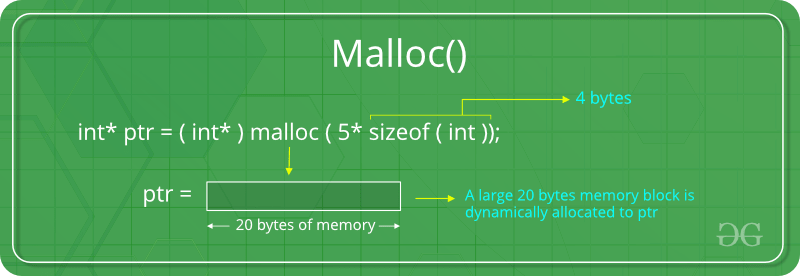If the space is insufficient, allocation fails and returns a NULL pointer.

Example:

 `#include ` `#include ` ` `  `int` `main() ` `{ ` ` `  `    ``// This pointer will hold the ` `    ``// base address of the block created ` `    ``int``* ptr; ` `    ``int` `n, i, sum = 0; ` ` `  `    ``// Get the number of elements for the array ` `    ``n = 5; ` `    ``printf``(``"Enter number of elements: %d\n"``, n); ` ` `  `    ``// Dynamically allocate memory using malloc() ` `    ``ptr = (``int``*)``malloc``(n * ``sizeof``(``int``)); ` ` `  `    ``// Check if the memory has been successfully ` `    ``// allocated by malloc or not ` `    ``if` `(ptr == NULL) { ` `        ``printf``(``"Memory not allocated.\n"``); ` `        ``exit``(0); ` `    ``} ` `    ``else` `{ ` ` `  `        ``// Memory has been successfully allocated ` `        ``printf``(``"Memory successfully allocated using malloc.\n"``); ` ` `  `        ``// Get the elements of the array ` `        ``for` `(i = 0; i < n; ++i) { ` `            ``ptr[i] = i + 1; ` `        ``} ` ` `  `        ``// Print the elements of the array ` `        ``printf``(``"The elements of the array are: "``); ` `        ``for` `(i = 0; i < n; ++i) { ` `            ``printf``(``"%d, "``, ptr[i]); ` `        ``} ` `    ``} ` ` `  `    ``return` `0; ` `} `

Output:

```Enter number of elements: 5
Memory successfully allocated using malloc.
The elements of the array are: 1, 2, 3, 4, 5,
```
2. calloc()

“calloc” or “contiguous allocation” method is used to dynamically allocate the specified number of blocks of memory of the specified type. It initializes each block with a default value ‘0’.

Syntax:

```ptr = (cast-type*)calloc(n, element-size);

For Example:
ptr = (float*) calloc(25, sizeof(float));

This statement allocates contiguous space in memory
for 25 elements each with the size of float.
```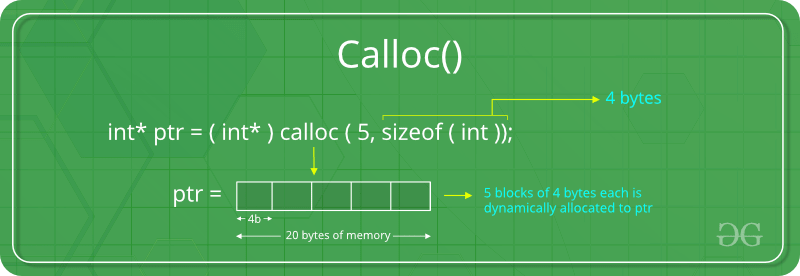If the space is insufficient, allocation fails and returns a NULL pointer.

Example:

 `#include ` `#include ` ` `  `int` `main() ` `{ ` ` `  `    ``// This pointer will hold the ` `    ``// base address of the block created ` `    ``int``* ptr; ` `    ``int` `n, i, sum = 0; ` ` `  `    ``// Get the number of elements for the array ` `    ``n = 5; ` `    ``printf``(``"Enter number of elements: %d\n"``, n); ` ` `  `    ``// Dynamically allocate memory using calloc() ` `    ``ptr = (``int``*)``calloc``(n, ``sizeof``(``int``)); ` ` `  `    ``// Check if the memory has been successfully ` `    ``// allocated by malloc or not ` `    ``if` `(ptr == NULL) { ` `        ``printf``(``"Memory not allocated.\n"``); ` `        ``exit``(0); ` `    ``} ` `    ``else` `{ ` ` `  `        ``// Memory has been successfully allocated ` `        ``printf``(``"Memory successfully allocated using calloc.\n"``); ` ` `  `        ``// Get the elements of the array ` `        ``for` `(i = 0; i < n; ++i) { ` `            ``ptr[i] = i + 1; ` `        ``} ` ` `  `        ``// Print the elements of the array ` `        ``printf``(``"The elements of the array are: "``); ` `        ``for` `(i = 0; i < n; ++i) { ` `            ``printf``(``"%d, "``, ptr[i]); ` `        ``} ` `    ``} ` ` `  `    ``return` `0; ` `} `

Output:

```Enter number of elements: 5
Memory successfully allocated using calloc.
The elements of the array are: 1, 2, 3, 4, 5,
```

c) ii) Write a program to multiply two matrices of dimension 3*3 and store the result in another matrix.

 `// C program to multiply two square matrices. ` ` `  `#include ` ` `  `const` `int` `MAX = 100; ` ` `  `// Function to print Matrix ` `void` `printMatrix(``int` `M[][MAX], ``int` `rowSize, ``int` `colSize) ` `{ ` `    ``for` `(``int` `i = 0; i < rowSize; i++) { ` `        ``for` `(``int` `j = 0; j < colSize; j++) ` `            ``printf``(``"%d "``, M[i][j]); ` ` `  `        ``printf``(``"\n"``); ` `    ``} ` `} ` ` `  `// Function to multiply two matrices A[][] and B[][] ` `void` `multiplyMatrix(``int` `row1, ``int` `col1, ``int` `A[][MAX], ` `                    ``int` `row2, ``int` `col2, ``int` `B[][MAX]) ` `{ ` `    ``int` `i, j, k; ` ` `  `    ``// Matrix to store the result ` `    ``int` `C[MAX][MAX]; ` ` `  `    ``// Check if multiplication is Possible ` `    ``if` `(row2 != col1) { ` `        ``printf``(``"Not Possible\n"``); ` `        ``return``; ` `    ``} ` ` `  `    ``// Multiply the two ` `    ``for` `(i = 0; i < row1; i++) { ` `        ``for` `(j = 0; j < col2; j++) { ` `            ``C[i][j] = 0; ` `            ``for` `(k = 0; k < row2; k++) ` `                ``C[i][j] += A[i][k] * B[k][j]; ` `        ``} ` `    ``} ` ` `  `    ``// Print the result ` `    ``printf``(``"\nResultant Matrix: \n"``); ` `    ``printMatrix(C, row1, col2); ` `} ` ` `  `// Driven Program ` `int` `main() ` `{ ` `    ``int` `row1, col1, row2, col2, i, j; ` `    ``int` `A[MAX][MAX], B[MAX][MAX]; ` ` `  `    ``// Read size of Matrix A from user ` `    ``printf``(``"Enter the number of rows of First Matrix: "``); ` `    ``scanf``(``"%d"``, &row1); ` `    ``printf``(``"%d"``, row1); ` `    ``printf``(``"\nEnter the number of columns of First Matrix: "``); ` `    ``scanf``(``"%d"``, &col1); ` `    ``printf``(``"%d"``, col1); ` ` `  `    ``// Read the elements of Matrix A from user ` `    ``printf``(``"\nEnter the elements of First Matrix: "``); ` `    ``for` `(i = 0; i < row1; i++) { ` `        ``for` `(j = 0; j < col1; j++) { ` `            ``printf``(``"\nA[%d][%d]: "``, i, j); ` `            ``scanf``(``"%d"``, &A[i][j]); ` `            ``printf``(``"%d"``, A[i][j]); ` `        ``} ` `    ``} ` ` `  `    ``// Read size of Matrix B from user ` `    ``printf``(``"\nEnter the number of rows of Second Matrix: "``); ` `    ``scanf``(``"%d"``, &row2); ` `    ``printf``(``"%d"``, row2); ` `    ``printf``(``"\nEnter the number of columns of Second Matrix: "``); ` `    ``scanf``(``"%d"``, &col2); ` `    ``printf``(``"%d"``, col2); ` ` `  `    ``// Read the elements of Matrix B from user ` `    ``printf``(``"\nEnter the elements of First Matrix: "``); ` `    ``for` `(i = 0; i < row2; i++) { ` `        ``for` `(j = 0; j < col2; j++) { ` `            ``printf``(``"\nB[%d][%d]: "``, i, j); ` `            ``scanf``(``"%d"``, &B[i][j]); ` `            ``printf``(``"%d"``, B[i][j]); ` `        ``} ` `    ``} ` ` `  `    ``// Print the Matrix A ` `    ``printf``(``"\n\nFirst Matrix: \n"``); ` `    ``printMatrix(A, row1, col1); ` ` `  `    ``// Print the Matrix B ` `    ``printf``(``"\nSecond Matrix: \n"``); ` `    ``printMatrix(B, row2, col2); ` ` `  `    ``// Find the product of the 2 matrices ` `    ``multiplyMatrix(row1, col1, A, row2, col2, B); ` ` `  `    ``return` `0; ` `} `

Output:

```Enter the number of rows of First Matrix: 2
Enter the number of columns of First Matrix: 3
Enter the elements of First Matrix:
A: 1
A: 2
A: 3
A: 4
A: 5
A: 6

Enter the number of rows of Second Matrix: 3
Enter the number of columns of Second Matrix: 2
Enter the elements of First Matrix:
B: 1
B: 2
B: 3
B: 4
B: 5
B: 6

First Matrix:
1 2 3
4 5 6

Second Matrix:
1 2
3 4
5 6

Resultant Matrix:
22 28
49 64
```

d) Convert the following numbers:
(i) (10101011101.011)2 = ()16

• (10101011101.011)2
= (55D.6)16
• (ii) (916.125)10 = ()4

• (916.125)10
= (32110.02)4
• (iii)(123)10 = ()2

• (123)10
= (1111011)2
• (iv) (574.32)8 = ()2

• (574.32)8
= (101111100.01101)2
• (v) (1011.10)2 = ()10

• (1011.10)2
= (11.5)10
• e) i) Write a program to print all prime numbers from 1 to 300.

 `// C program to check if a ` `// number is prime ` ` `  `#include ` ` `  `int` `main() ` `{ ` `    ``int` `n = 1, i, flag = 1; ` ` `  `    ``for` `(n = 1; n <= 300; n++) { ` `        ``flag = 1; ` `        ``// Iterate from 2 to n/2 ` `        ``for` `(i = 2; i <= n / 2; i++) { ` ` `  `            ``// If n is divisible by any number between ` `            ``// 2 and n/2, it is not prime ` `            ``if` `(n % i == 0) { ` `                ``flag = 0; ` `                ``break``; ` `            ``} ` `        ``} ` ` `  `        ``if` `(flag == 1) { ` `            ``printf``(``"\n%d is a prime number"``, n); ` `        ``} ` `    ``} ` `    ``return` `0; ` `} `

Output:

```1 is a prime number
2 is a prime number
3 is a prime number
5 is a prime number
7 is a prime number
11 is a prime number
13 is a prime number
17 is a prime number
19 is a prime number
23 is a prime number
29 is a prime number
31 is a prime number
37 is a prime number
41 is a prime number
43 is a prime number
47 is a prime number
53 is a prime number
59 is a prime number
61 is a prime number
67 is a prime number
71 is a prime number
73 is a prime number
79 is a prime number
83 is a prime number
89 is a prime number
97 is a prime number
101 is a prime number
103 is a prime number
107 is a prime number
109 is a prime number
113 is a prime number
127 is a prime number
131 is a prime number
137 is a prime number
139 is a prime number
149 is a prime number
151 is a prime number
157 is a prime number
163 is a prime number
167 is a prime number
173 is a prime number
179 is a prime number
181 is a prime number
191 is a prime number
193 is a prime number
197 is a prime number
199 is a prime number
211 is a prime number
223 is a prime number
227 is a prime number
229 is a prime number
233 is a prime number
239 is a prime number
241 is a prime number
251 is a prime number
257 is a prime number
263 is a prime number
269 is a prime number
271 is a prime number
277 is a prime number
281 is a prime number
283 is a prime number
293 is a prime number
```

e) ii) Any year is input through the keyboard. Write a program to determine whether the year is a leap year or not.

 `// C program to check if a given ` `// year is leap year or not ` ` `  `#include ` `#include ` ` `  `bool` `checkYear(``int` `year) ` `{ ` `    ``// If a year is multiple of 400, ` `    ``// then it is a leap year ` `    ``if` `(year % 400 == 0) ` `        ``return` `true``; ` ` `  `    ``// Else If a year is multiple of 100, ` `    ``// then it is not a leap year ` `    ``if` `(year % 100 == 0) ` `        ``return` `false``; ` ` `  `    ``// Else If a year is multiple of 4, ` `    ``// then it is a leap year ` `    ``if` `(year % 4 == 0) ` `        ``return` `true``; ` `    ``return` `false``; ` `} ` ` `  `// driver code ` `int` `main() ` `{ ` `    ``int` `year; ` ` `  `    ``// Get the year ` `    ``printf``(``"Enter the year: "``); ` `    ``scanf``(``"%d"``, &year); ` `    ``printf``(``"%d"``, year); ` ` `  `    ``// Check if year is leap year ` `    ``checkYear(year) ` `        ``? ``printf``(``"\nLeap Year"``) ` `        ``: ``printf``(``"\nNot a Leap Year"``); ` `    ``return` `0; ` `} `

Output:

```Enter the year: 2018
Not a Leap Year
```

f) What do you mean by parameter passing? Discuss various types of parameter passing mechanism in C with examples.

Assigning values to the variables defined in the function definition in runtime is known as parameter passing. There are different ways in which parameter data can be passed into and out of methods and functions. Let us assume that a function B() is called from another function A(). In this case A is called the “caller function” and B is called the “called function or callee function”. Also, the arguments which A sends to B are called actual arguments and the parameters of B are called formal arguments.

Important methods of Parameter Passing

1. Pass By Value : This method uses in-mode semantics. Changes made to formal parameter do not get transmitted back to the caller. Any modifications to the formal parameter variable inside the called function or method affect only the separate storage location and will not be reflected in the actual parameter in the calling environment. This method is also called as call by value.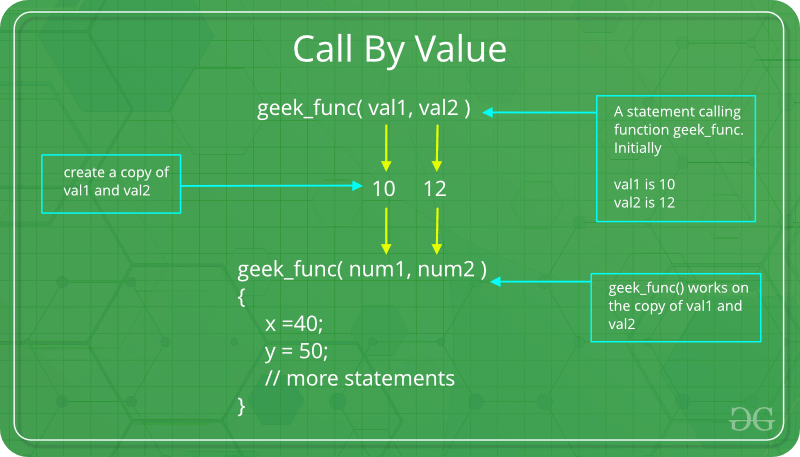`// C program to illustrate ` `// call by value ` `#include ` ` `  `void` `func(``int` `a, ``int` `b) ` `{ ` `    ``a += b; ` `    ``printf``(``"In func, a = %d b = %d\n"``, a, b); ` `} ` `int` `main(``void``) ` `{ ` `    ``int` `x = 5, y = 7; ` ` `  `    ``// Passing parameters ` `    ``func(x, y); ` `    ``printf``(``"In main, x = %d y = %d\n"``, x, y); ` `    ``return` `0; ` `} `

Output:

```In func, a = 12 b = 7
In main, x = 5 y = 7
```

Languages like C, C++, Java support this type of parameter passing. Java in fact is strictly call by value.
Shortcomings:

• Inefficiency in storage allocation
• For objects and arrays, the copy semantics are costly
2. Pass by reference(aliasing) : This technique uses in/out-mode semantics. Changes made to formal parameter do get transmitted back to the caller through parameter passing. Any changes to the formal parameter are reflected in the actual parameter in the calling environment as formal parameter receives a reference (or pointer) to the actual data. This method is also called as <em>call by reference. This method is efficient in both time and space.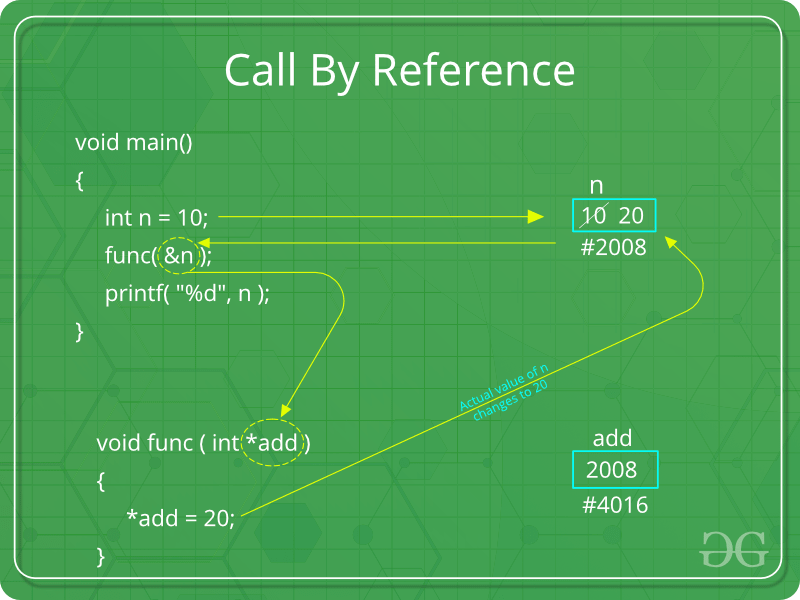`// C program to illustrate ` `// call by reference ` `#include ` ` `  `void` `swapnum(``int``* i, ``int``* j) ` `{ ` `    ``int` `temp = *i; ` `    ``*i = *j; ` `    ``*j = temp; ` `} ` ` `  `int` `main(``void``) ` `{ ` `    ``int` `a = 10, b = 20; ` ` `  `    ``// passing parameters ` `    ``swapnum(&a, &b); ` ` `  `    ``printf``(``"a is %d and b is %d\n"``, a, b); ` `    ``return` `0; ` `} `

Output:

```a is 20 and b is 10
```

g) i) Define data types in C. Discuss primitive data types in terms of memory occupied, format specifier and range.

Each variable in C has an associated data type. Each data type requires different amounts of memory and has some specific operations which can be performed over it. Let us briefly describe them one by one:

Following are the examples of some very common data types used in C:

• char: The most basic data type in C. It stores a single character and requires a single byte of memory in almost all compilers.
• int: As the name suggests, an int variable is used to store an integer.
• float: It is used to store decimal numbers (numbers with floating point value) with single precision.
• double: It is used to store decimal numbers (numbers with floating point value) with double precision.

Different data types also have different ranges upto which they can store numbers. These ranges may vary from compiler to compiler. Below is list of ranges along with the memory requirement and format specifiers on 32 bit gcc compiler.

Data Type Memory (bytes) Range Format Specifier
short int 2 -32, 768 to 32, 767 %hd
unsigned short int 2 0 to 65, 535 %hu
unsigned int 4 0 to 4, 294, 967, 295 %u
int 4 -2, 147, 483, 648 to 2, 147, 483, 647 %d
long int 4 -2, 147, 483, 648 to 2, 147, 483, 647 %ld
unsigned long int 4 0 to 4, 294, 967, 295 %lu
long long int 8 -(2^63) to (2^63)-1 %lld
unsigned long long int 8 0 to 18, 446, 744, 073, 709, 551, 615 %llu
signed char 1 -128 to 127 %c
unsigned char 1 0 to 255 %c
float 4 %f
double 8 %lf
long double 12 %Lf

g) ii) Write a program to print the following pattern:

```A
AB
ABC
ABCD
ABCDE
ABCDEF
```

 `#include ` ` `  `int` `main() ` `{ ` ` `  `    ``char` `ch = ``'A'``; ` `    ``int` `i, j; ` ` `  `    ``for` `(i = 0; i < 5; i++) { ` `        ``for` `(j = 0; j <= i; j++) { ` `            ``printf``(``"%c"``, ch + j); ` `        ``} ` ` `  `        ``printf``(``"\n"``); ` `    ``} ` ` `  `    ``return` `0; ` `} `

Output:

```A
AB
ABC
ABCD
ABCDE
```

h) List out various file operations and modes in C. Write a program to copy the content from one file to another file.
File opening modes in C:

• “r” – Searches file. If the file is opened successfully fopen( ) loads it into memory and sets up a pointer which points to the first character in it. If the file cannot be opened fopen( ) returns NULL.
• “w” – Searches file. If the file exists, its contents are overwritten. If the file doesn’t exist, a new file is created. Returns NULL, if unable to open file.
• “a” – Searches file. If the file is opened successfully fopen( ) loads it into memory and sets up a pointer that points to the last character in it. If the file doesn’t exist, a new file is created. Returns NULL, if unable to open file.
• “r+” – Searches file. If is opened successfully fopen( ) loads it into memory and sets up a pointer which points to the first character in it. Returns NULL, if unable to open the file.
• “w+” – Searches file. If the file exists, its contents are overwritten. If the file doesn’t exist a new file is created. Returns NULL, if unable to open file.
• “a+” – Searches file. If the file is opened successfully fopen( ) loads it into memory and sets up a pointer which points to the last character in it. If the file doesn’t exist, a new file is created. Returns NULL, if unable to open file.

C program to copy contents of one file to another file:

 `#include ` `#include // For exit() ` ` `  `int` `main() ` `{ ` `    ``FILE` `*fptr1, *fptr2; ` `    ``char` `filename, c; ` ` `  `    ``printf``(``"Enter the filename to open for reading \n"``); ` `    ``scanf``(``"%s"``, filename); ` ` `  `    ``// Open one file for reading ` `    ``fptr1 = ``fopen``(filename, ``"r"``); ` `    ``if` `(fptr1 == NULL) { ` `        ``printf``(``"Cannot open file %s \n"``, filename); ` `        ``exit``(0); ` `    ``} ` ` `  `    ``printf``(``"Enter the filename to open for writing \n"``); ` `    ``scanf``(``"%s"``, filename); ` ` `  `    ``// Open another file for writing ` `    ``fptr2 = ``fopen``(filename, ``"w"``); ` `    ``if` `(fptr2 == NULL) { ` `        ``printf``(``"Cannot open file %s \n"``, filename); ` `        ``exit``(0); ` `    ``} ` ` `  `    ``// Read contents from file ` `    ``c = ``fgetc``(fptr1); ` `    ``while` `(c != EOF) { ` `        ``fputc``(c, fptr2); ` `        ``c = ``fgetc``(fptr1); ` `    ``} ` ` `  `    ``printf``(``"\nContents copied to %s"``, filename); ` ` `  `    ``fclose``(fptr1); ` `    ``fclose``(fptr2); ` `    ``return` `0; ` `} `

Output:

```Enter the filename to open for reading
Cannot open file
```

Output:

```Enter the filename to open for reading
a.txt
Enter the filename to open for writing
b.txt
Contents copied to b.txt
```

3. My Personal Notes arrow_drop_upCheck out this Author's contributed articles.

If you like GeeksforGeeks and would like to contribute, you can also write an article using contribute.geeksforgeeks.org or mail your article to contribute@geeksforgeeks.org. See your article appearing on the GeeksforGeeks main page and help other Geeks.

Please Improve this article if you find anything incorrect by clicking on the "Improve Article" button below.

Article Tags :

Be the First to upvote.

Please write to us at contribute@geeksforgeeks.org to report any issue with the above content.